# Quiz 2: Linear Programming: Model Formulation and Graphical Solution

Linear Programming is a mathematical modeling technique containing linear relationships that represent an institution's objectives and resource and/or material constraints. This technique consists of 3 elements: 1. Decision variables: they are symbols that denote the activities such as x 1, x 2, x 3 and so on. 2. Objective function: they represent the relationships between the variables subject to the constraints. 3. Model constraints: they represent the constraints on the variables. There are 2 decision variables.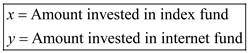Objective function : The objective is to maximize the annual return on the investment made. Average annual returns of the index fund is 17%, whereas average annual returns of the Internet fund is 28%. Price per share for index fund is \$175 and for internet fund is \$208. Thus, the objective function becomes: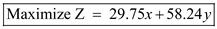The objective function is subject to the following constraints: Constraint 1: Funds available to invest are \$120,000. Thus, the first constraint is: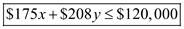Constraint 2: Proportion of amount invested in the index fund should be at least one third as compared to the Internet fund. Thus, the second constraint is:Constraint 3: Not more than twice the amount in the Internet fund should be invested in the index fund. Thus, the third constraint is:Constraint 4: Non-negativity constraint. Both decision variables should be non-negative i.e. either 0 or more than 0.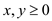Thus, the linear programming model is formulated for person A and is summarized below:Now, solve the linear programming model using graphical analysis method as shown below: Convert the inequalities into equalities as shown below: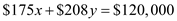…… (1)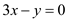…… (2)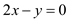…… (3) Now, put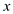= 0 in equation (1) would give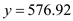. Again, putting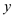= 0 in equation (1) would give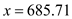. Hence, two coordinates are obtained using this technique i.e. (0, 576.92) and (685.71, 0) for equation 1. Similarly, coordinates for the remaining two equations are obtained. Plot the three coordinates on the graph and connect the points by a line to get the graphical representation of each constraint. The graphical solution of the linear programming model is as follows: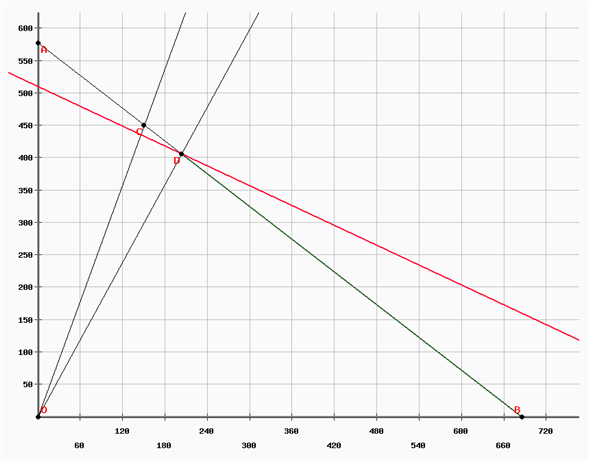The corner points and the values of the objective function are given as follows: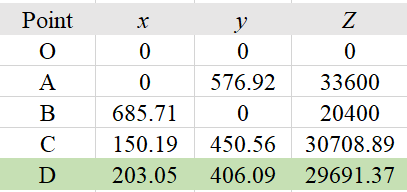Thus, the optimal solution for the linear programming model is obtained. Person A should invest \$203.05 and \$406.09 in index fund and internet fund respectively to maximize its annual return to \$29,691.37. Suppose, the linear programming model gets modified to following:Compute the coordinates for both constraints as done earlier and plot them on the graph and connect the points by a line to get the graphical representation of each constraint. The graphical solution of the linear programming model is as follows: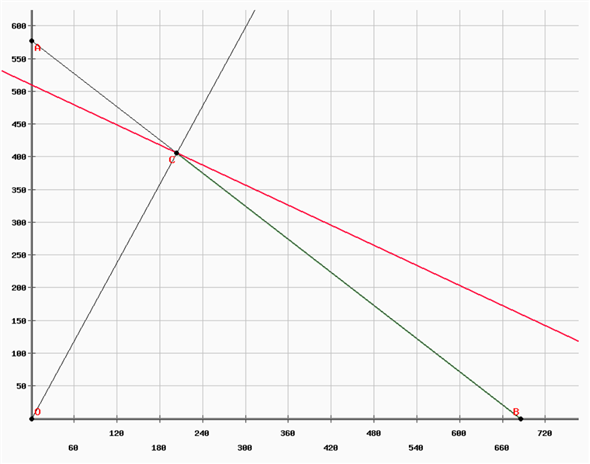The corner points and the values of the objective function are given as follows: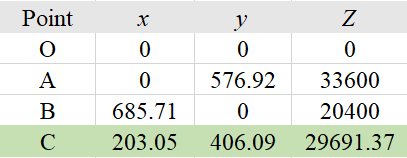One can see that there is no change in the optimal solution despite eliminating the restriction that the proportion of amount invested in the index fund should be at least one third as compared to the Internet fund. Suppose, the linear programming model gets modified to following:Compute the coordinates for both constraints as done earlier and plot them on the graph and connect the points by a line to get the graphical representation of each constraint. The graphical solution of the linear programming model is as follows: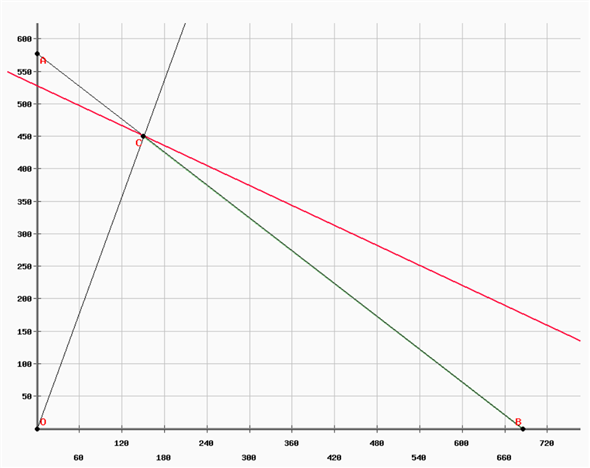The corner points and the values of the objective function are given as follows: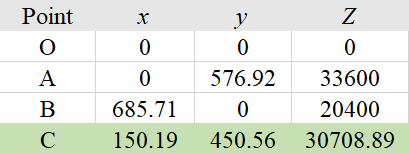When the restriction that not more than twice the amount in the Internet fund should be invested in the index fund is eliminated, the optimal solution changes. Person A should invest \$150.19 and \$4050.56 in index fund and internet fund respectively to maximize its annual return to \$30,708.89. If person A gets \$1 more to invest, the linear programming model changes to:Solving the model using graphical analysis, the graph and corner points are as follows: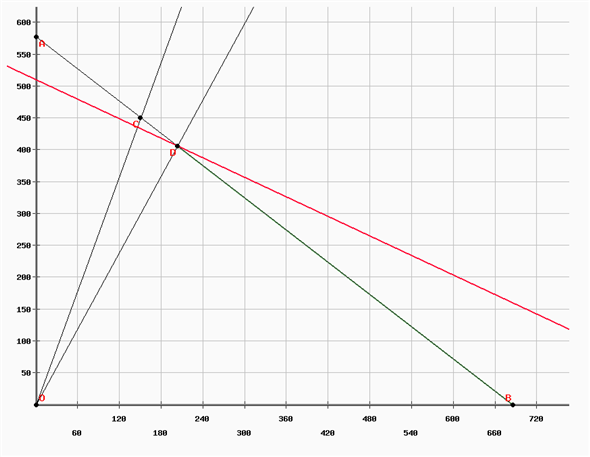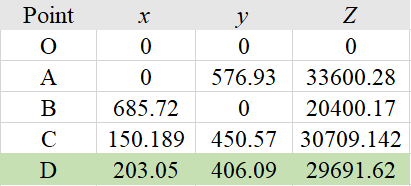Adding \$1 more to invest increases the annual returns from \$29,691.37 to \$29,691.62 i.e. a difference of \$0.25. If person A gets \$2 more to invest, the linear programming model changes to:Solving the model using graphical analysis, the graph and corner points are as follows: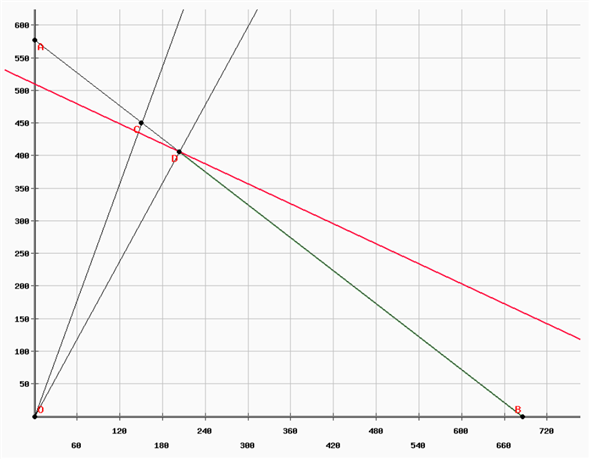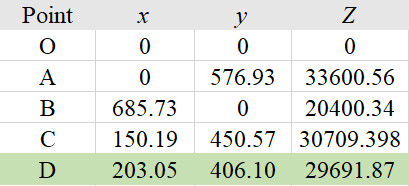Adding \$2 more to invest increases the annual returns from \$29,691.37 to \$29,691.87 i.e. a difference of \$0.50. If person A gets \$3 more to invest, the linear programming model changes to:Solving the model using graphical analysis, the graph and corner points are as follows: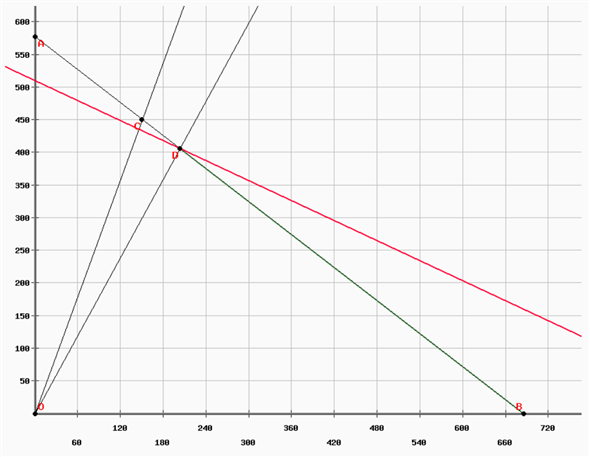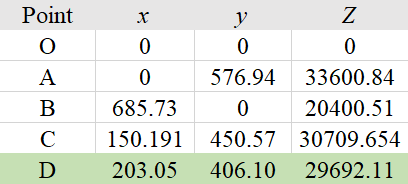Adding \$3 more to invest increases the annual returns from \$29,691.37 to \$29,692.11 i.e. a difference of \$0.74. One can see that by adding \$1 more to invest, the annual returns increase by approximately \$0.25. Thus, with for every dollar of increase in investment, the annual return on her investment increases by \$0.25.
The horizontal and vertical distance of each sector is considered to be x and y, respectively. Objective of the problem is to minimize the total time required by the police department in responding to the calls. Given,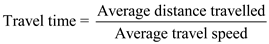Therefore, objective of the problem can be mathematically represented as shown below:Average travel speeds for patrol cars in horizontal and vertical directions are 20 miles per hour and 15 miles per hour respectively. Now, write down the constraints of the linear programming problem as shown below: Constraint 1: Total perimeter of a petrol sector should not be less than 5 miles. Perimeter of each block is calculated using given formula: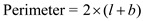…… (Since, each block is rectangular in shape) Therefore,Here,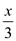and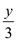are the average dimension of each sector in horizontal and vertical direction. Constraint 2: Total perimeter of a petrol sector should not be greater than 12 miles.Constraint 3: The distance travelled in vertical direction should be at least 1.5 times the horizontal distance.Constraint 4: Non-negativity constraint for the distance travelled in horizontal and vertical directions.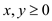Hence, the linear programming problem for the given data is as follows: Objective: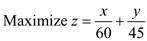Constraints:Now, solve the linear programming model using graphical analysis method as shown below: Convert the inequalities into equalities as shown below: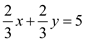…… (1)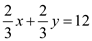…… (2)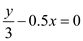…… (3) Now, put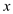= 0 in equation (1). It would give the following results: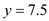Again, put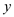= 0 in equation (1). It would give the following results: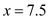Hence, two points are obtained using this technique i.e. (0, 7.5) and (7.5, 0). Similarly, find other points from the remaining equations. Prepare a graph by joining different points obtained from solving each equation, as shown below: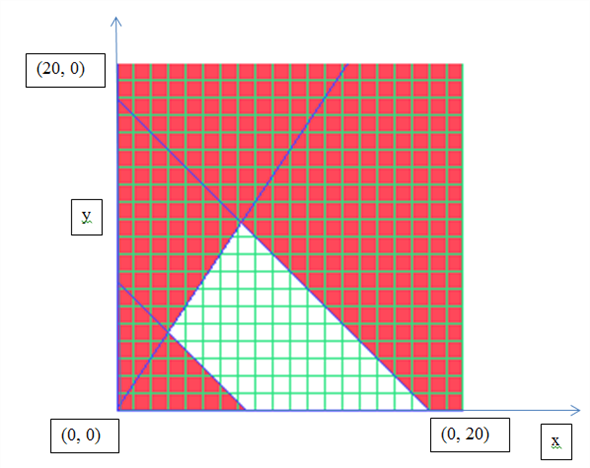The white shaded region represents the area of optimal solution. Calculate the optimal solution using excel solver as shown below: Prepare an excel sheet as follows: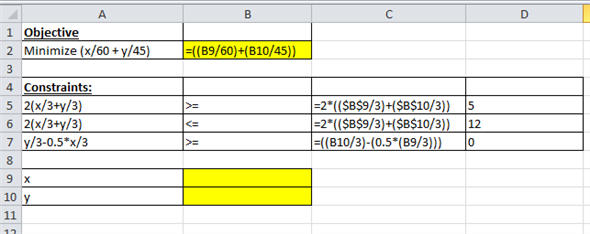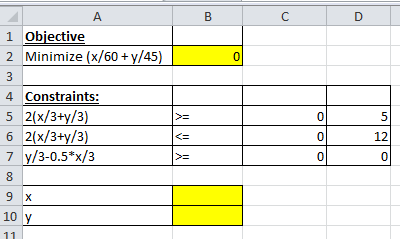Access "Excel solver" from menu bar and enter the following values in the pop-up window: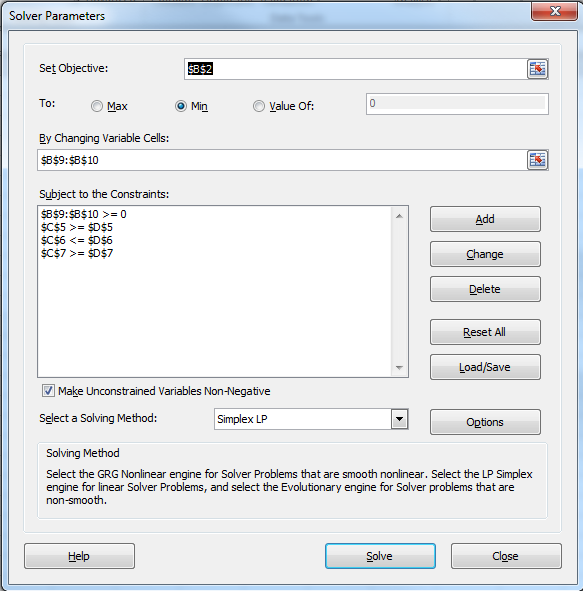Click on "Solve" option. Then, following pop up would appear:Click on "OK" button. It would give the following values: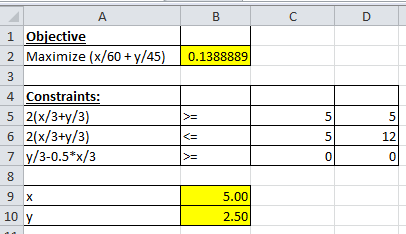Hence, it is concluded that the minimum time required by the petrol cars would be 0.139 hour , if they reduce the horizontal and vertical dimension of a block to 5 miles and 2.5 miles , respectively.
(a) Formulate the linear programming equations by following the steps as shown below: Step1: Identify the Decision Variables: The first step is to develop the variables, as per the question there two variables- the number of cakes to be baked and the number of breads to be prepared in the oven. They are the two variables as shown below: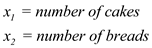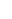Step2: Formulate the Objective Function Next step is to develop the objective equation as shown below:(b) Step3: Draw the graphical solution for the problem as shown below: Each of the equation needs to be drawn as shown below: For the first equation: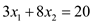points are: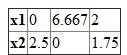For the second equation: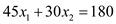points are: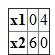Plot the equation's point on the graph as shown below: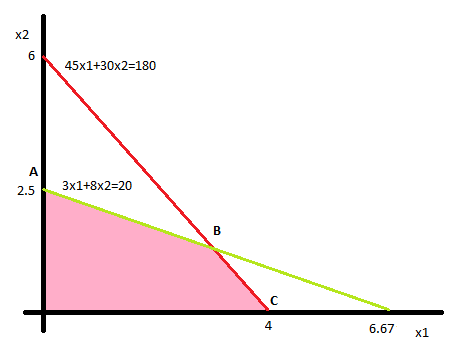The pink region reflects the feasible are for person T to prepare the number of cakes and breads. The corner points for the optimal x1 and x2 are shown as below: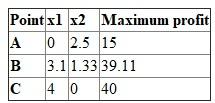Thus, profits are maximized at \$40 when person T prepares 4 cakes and zero breads.